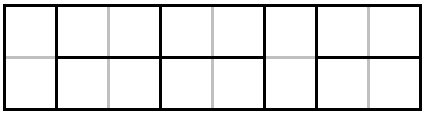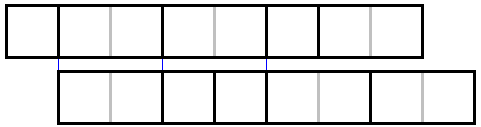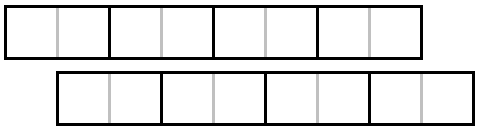# Fibonacci Tilings

Fibonacci numbers $\{F_{n}, n \ge 0\}$ satisfy the recurrence relation

(1)

$F_{n+2} = F_{n+1} + F_{n},$

along with the initial conditions $F_{1} = 1$ and $F_{0} = 0.$

The Fibonacci name has been attached to the sequence $0, 1, 1, 2, 3, 5, \ldots$ due to the inclusion in his 1202 book Liber Abaci of a rabbit reproduction puzzle: under certain constraints the rabbit population at discrete times is given exactly by that sequence. As naturally, the sequence is simulated by counting the tilings with dominoes of a $2\times n$ board:A tiling of a $2\times n$ board may end with two horizontal dominoes or a single vertical domino:In the former case, it's an extension of a tiling of a $2\times (n-2)$ board; in the latter case, it's an extension of a tiling of a $2\times (n-1).$ If $T_{n}$ denotes the number of domino tilings of a $2\times n$ board, then clearly

$T_{n} = T_{n-2} + T_{n-1}$

which is the same recurrence relation that is satisfied by the Fibonacci sequence. By a direct verification, $T_{1} = 1,$ $T_{2} = 2,$ $T_{3} = 3,$ $T_{4} = 5,$ etc., which shows that $\{T_{n}\}$ is nothing but a shifted Fibonacci sequence. If we define, $T_{0} = 1,$ as there is only one way to do nothing; and $T_{-1} = 0,$ because there are no boards with negative side lengths, then $F_{n} = T_{n-1},$ for $n \ge 0.$

The domino tilings are extensively used in Graham, Knuth, Patashnik and by Zeitz. Benjamin & Quinn economize by considering only an upper $1\times n$ portion of the board (and its tilings). This means tiling a $1\times n$ board with $1\times 1$ and $1\times 2$ pieces.

I'll use Benjamin & Quinn's frugal tilings to prove Cassini's Identity

$F_{n+1}\cdot F_{n+1} - F_{n}\cdot F_{n+2} = (-1)^{n}.$

In terms of the tilings, I want to prove that $T_{n}\cdot T_{n} - T_{n-1}\cdot T_{n+1} = (-1)^{n}$.

The meaning of the term $T_{n}\cdot T_{n}$ is obvious: this is the number of ways to tile two $1\times n$ boards where the tilings of the two boards are independent of each other. Similarly, $T_{n-1}T_{n+1}$ is the number of ways to tile two boards: one $1\times (n-1)$ and one $1\times(n+1).$ Now, the task is to retrieve the relation between the two numbers annunciated by Cassini's identity.

Our setup consists of two $1\times n$ boards:with the bottom board shifted one square to the right:The tilings of the two boards may or may not have a fault line. A fault line is a line on the two boards at which the two tilings are breakable. For example, the tilings below have three fault lines:The trick is now to swap tails: the pieces of the two tilings (along with the boards) after the last fault line:Since the bottom board has been shifted just one square, the swap produces one tiling of a $1\times (n+1)$ - the top board in the diagram - and one tiling of a $1\times (n-1)$ board - the bottom board in the diagram. Note that the old faults have been preserved and no new faults have been introduced.

Thus, in the presence of faults, there is a 1-1 correspondence between two $n$-tilings $(T_{n})$ and a pair of $(n-1)$- and $(n+1)$-tilings. The time is to account for the faultless combinations, if any.

But there are. Any $1\times 1$ square induces a fault. This leaves exactly two faultless tilings. If $n$ is odd, both $n-1$ and $n+1$ are even, there is a unique pair of $(n-1)-$ and $(n+1)$-tilings:If $n$ is even, there is a unique $n$-tiling that, when shifted, generates no fault lines:### References

1. A. T. Benjamin, J. J. Quinn, Proofs That Really Count: The Art of Combinatorial Proof, MAA, 2003
2. R. Graham, D. Knuth, O. Patashnik, Concrete Mathematics, 2nd edition, Addison-Wesley, 1994.
3. D. Singmaster, Problems for Metagrobologists, World Scientific, 2016, #183
4. P. Zeitz, The Art and Craft of Problem Solving, John Wiley & Sons, 1999• The Basic Rules of Counting
• Combinatorial Proofs
• The Inclusion-Exclusion Principle
• Inclusion-Exclusion Principle: an Example
• Ramsey's Theorem
• Coloring Points in the Plane
• Probabilities
• Example: A Poker Hand
•